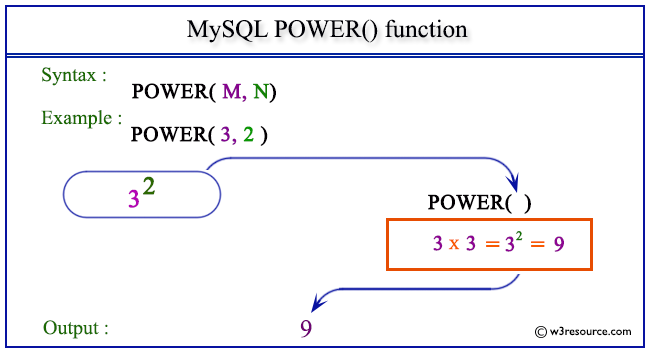# MySQL POWER() function

## POWER() function

MySQL POWER() returns the value of a number raised to the power of another number. The synonym of POWER() is POW().

Syntax:

```POWER(M,N);
```

Arguments

Name Description
M A number which is the base of the exponentiation.
N A number which is the exponent of the exponentiation.

Syntax Diagram:MySQL Version: 5.6

Pictorial presentation of MySQL POWER() functionExample of MySQL POWER() function

Code:

``````SELECT POWER(3, 2);
```
```

Explanation

The above MySQL statement returns the value of 32, i.e. 9.

Sample Output:

```mysql> SELECT POWER(3, 2);
+-------------+
| POWER(3, 2) |
+-------------+
|           9 |
+-------------+
1 row in set (0.00 sec)
```

Example : POWER() function with negative value

Code:

``````SELECT POWER(4,-2);
```
```

Explanation

The above MySQL statement returns the value of 4-2, i.e. 0.0625.

Sample Output:

```mysql> SELECT POWER(4,-2);
+-------------+
| POWER(4,-2) |
+-------------+
|      0.0625 |
+-------------+
1 row in set (0.00 sec)
```

All Mathematical FunctionsPrevious: POW()